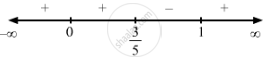Share

# F(X) = X3 (X − 1)2 . - Mathematics

#### Question

f(x) = x3  (x $-$ 1).

#### Solution

$\text { Given }: f\left( x \right) = x^3 \left( x - 1 \right)^2$

$\Rightarrow f'\left( x \right) = 3 x^2 \left( x - 1 \right)^2 + 2 x^3 \left( x - 1 \right)$

$\text { For a local maximum or a local minimum, we must have }$

$f'\left( x \right) = 0$

$\Rightarrow 3 x^2 \left( x - 1 \right)^2 + 2 x^3 \left( x - 1 \right) = 0$

$\Rightarrow x^2 \left( x - 1 \right)\left\{ 3x - 3 + 2x \right\} = 0$

$\Rightarrow x^2 \left( x - 1 \right)\left( 5x - 3 \right) = 0$

$\Rightarrow x = 0, 1, \frac{3}{5}$Since f '(x) changes from negative to positive when x increases through 1, x = 1 is the point of local minima.

The local minimum value of  f (x)  at x = 1 is given by $\left( 1 \right)^3 \left( 1 - 1 \right)^2 = 0$

Since f '(x) changes from positive to negative when x increases through $\frac{3}{5}$, x = $\frac{3}{5}$ is the point of local maxima.

The local minimum value of  f (x) at x =  $\frac{3}{5}$ is given by $\left( \frac{3}{5} \right)^3 \left( \frac{3}{5} - 1 \right)^2 = \frac{27}{125} \times \frac{4}{25} = \frac{108}{3125}$
Sincef '(x) does not change from positive as x increases through 0, x = 0 is a point of inflexion.

#### Notes

The solution in the book is incorrect. The solution here is created according to the question given in the book.

Is there an error in this question or solution?